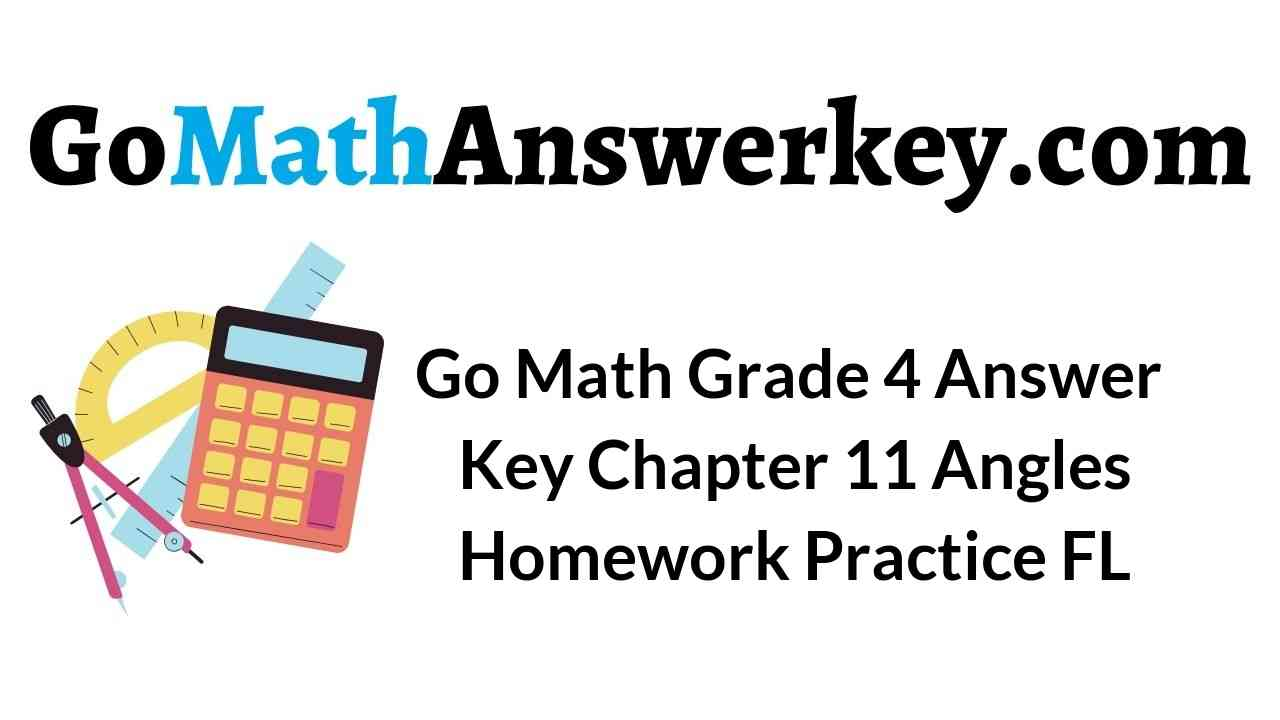# Go Math Grade 4 Answer Key Homework Practice FL Chapter 11 Angles

## Go Math Grade 4 Answer Key Homework Practice FL Chapter 11 Angles

You can succeed in your academics as well as sharpen your math skills by solving Go Math Grade 4 Answer Key Homework Practice FL Chapter 11 Angles. Practicing from HMH Grade 4 Go Math Solution Key you will have numerous benefits. Tap on the link and start solving the answers for the homework practice questions.

Lesson: 1 – Angles and Fractional Parts of a Circle

Lesson: 2 – Degrees

Lesson: 3 – Measure and Draw Angles

Lesson: 4 – Join and Separate Angles

Lesson: 5 – Problem Solving Unknown Angle Measures

Lesson: 11.1

### Common Core – Angles – Page No. 207

Angles and Fractional Parts of a Circle

Tell what fraction of the circle the shaded angle represents.

Question 1.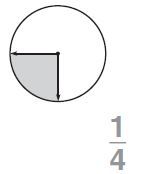Explanation:
By seeing the above figure we can say that the fraction of the shaded part is $$\frac{1}{4}$$

Question 2.$$\frac{□}{□}$$

Answer: $$\frac{1}{2}$$

Explanation:
Half of the circle is shaded in the above figure. The fraction of the shaded part is $$\frac{1}{2}$$.

Question 3.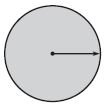$$\frac{□}{□}$$

Answer: $$\frac{1}{1}$$

Explanation:
The above circle is completely shaded. So, the fraction of the shaded part is $$\frac{1}{1}$$.

Tell whether the angle on the circle shows a $$\frac{1}{4}, \frac{1}{2}, \frac{3}{4}$$, or 1 full turn clockwise or counterclockwise.

Question 4.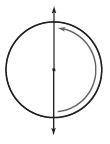$$\frac{□}{□}$$

Answer: $$\frac{1}{2}$$

Explanation:
By seeing the above figure we can say that the circle turns $$\frac{1}{2}$$ counterclockwise.

Question 5.$$\frac{□}{□}$$

Answer: $$\frac{3}{4}$$

Explanation:
By seeing the above figure we can say that the circle turns $$\frac{3}{4}$$ counterclockwise.

Question 6.__________

Answer: $$\frac{1}{1}$$

Explanation:
The above circle turns $$\frac{1}{1}$$ counterclockwise.

Problem Solving

Question 7.
Shelley exercised for 15 minutes. Describe the turn the minute hand made.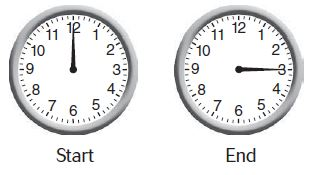Type below:
__________

Answer: $$\frac{1}{4}$$ Clockwise
The minute hand is on 3 which means the minute hand made $$\frac{1}{4}$$ Clockwise.

Question 8.
Mark took 30 minutes to finish lunch. Describe the turn the minute hand made.Type below:
__________

Answer: $$\frac{1}{2}$$ Clockwise
The minute hand is on 6 which means the minute hand made $$\frac{1}{2}$$ Clockwise.

### Common Core – Angles – Page No. 208

Lesson Check

Question 1.
What fraction of the circle does the shaded angle represent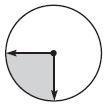Options:
a. $$\frac{1}{1}$$ or 1
b. $$\frac{3}{4}$$
c. $$\frac{1}{2}$$
d. $$\frac{1}{4}$$

Answer: $$\frac{1}{4}$$

Explanation:
The above figure shows that the fraction of the shaded part is $$\frac{1}{4}$$
Thus the correct answer is option D.

Question 2.
Which describes the turn shown below?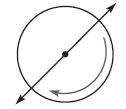Options:
a. $$\frac{1}{4}$$ turn clockwise
b. $$\frac{1}{2}$$ turn clockwise
c. $$\frac{1}{4}$$ turn counterclockwise
d. $$\frac{1}{2}$$ turn counterclockwise

Answer: $$\frac{1}{2}$$ turn clockwise

Explanation:
The circle made half turn. The fraction of the circle is $$\frac{1}{2}$$ turn clockwise.
Thus the correct answer is option B.

Spiral Review

Question 3.
Which shows $$\frac{2}{3}$$ and $$\frac{3}{4}$$ written as a pair of fractions with a common denominator?
Options:
a. $$\frac{2}{3} \text { and } \frac{4}{3}$$
b. $$\frac{6}{9} \text { and } \frac{6}{8}$$
c. $$\frac{2}{12} \text { and } \frac{3}{12}$$
d. $$\frac{8}{12} \text { and } \frac{9}{12}$$

Answer: $$\frac{8}{12} \text { and } \frac{9}{12}$$

Explanation:
Given the fraction $$\frac{2}{3}$$ and $$\frac{3}{4}$$
LCM of 3 and 4 is 12
$$\frac{2}{3}$$ × $$\frac{4}{4}$$ = $$\frac{8}{12}$$
$$\frac{3}{4}$$ × $$\frac{3}{3}$$ = $$\frac{9}{12}$$
Thus the correct answer is option D.

Question 4.
Raymond bought $$\frac{3}{4}$$ of a dozen rolls. How many rolls did he buy?
Options:
a. 3
b. 6
c. 7
d. 9

Explanation:
Given that,
Raymond bought $$\frac{3}{4}$$ of a dozen rolls.
$$\frac{3}{4}$$ × 12 = 3 × 3 = 9
Thus the correct answer is option D.

Question 5.
Which of the following lists all the factors of 18?
Options:
a. 1, 2, 4, 9, 18
b. 1, 2, 3, 6, 9, 18
c. 2, 3, 6, 9
d. 1, 3, 5, 9, 18

Answer: 1, 2, 3, 6, 9, 18

Explanation:
The factors of 18 are
1 × 18 = 18
2 × 9 = 18
3 × 6 = 18
6 × 3 = 18
9 × 2 = 18
18 × 1 = 18
The factors are 1, 2, 3, 6, 9, 18.
Thus the correct answer is option B.

Question 6.
Jonathan rode 1.05 miles on Friday, 1.5 miles on Saturday, 1.25 miles on Monday, and 1.1 miles on Tuesday. On which day did he ride the shortest distance?
Options:
a. Monday
b. Tuesday
c. Friday
d. Saturday

Explanation:
Given that,
Jonathan rode 1.05 miles on Friday, 1.5 miles on Saturday, 1.25 miles on Monday, and 1.1 miles on Tuesday.
1.05 < 1.1 < 1.5
Thus the shortest distance is 1.05 miles that is on Friday.
Thus the correct answer is option C.

### Common Core – Angles – Page No. 209

Degrees

Tell the measure of the angle in degrees.

Question 1.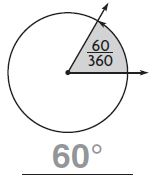60°

Question 2.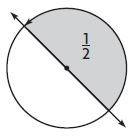_____°

Explanation:
The complete angle of the circle is 360°
The above circle made half turn
1/2 × 360° = 180°

Question 3._____°

Explanation:
The complete angle of the circle is 360°
The above circle made 1/4 turn.
1/4 × 360° = 90°

Classify the angle. Write acute, obtuse, right, or straight.

Question 4.__________

Explanation:
The acute angle is the small angle which is less than 90°. The above angle is 25° which is less than 90°. Thus the above angle is an acute angle.

Question 5.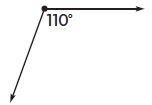__________

Explanation:
An obtuse angle has a measurement greater than 90 degrees but less than 180 degrees. The above angle is 110° which is greater than 90° and less than 180°. Thus the angle of the above figure is an obtuse angle.

Question 6.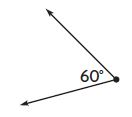__________

Explanation:
The acute angle is the small angle which is less than 90°. The above angle is 60° which is less than 90°. Thus the above angle is an acute angle.

Classify the triangle. Write acute, obtuse, or right.

Question 7.__________

Explanation:
In geometry and trigonometry, a right angle is an angle of exactly 90°, corresponding to a quarter turn.

Question 8.__________

Explanation:
An obtuse angle has a measurement greater than 90 degrees but less than 180 degrees. The above angle is 110° which is greater than 90° and less than 180°. Thus the angle of the above figure is an obtuse angle.

Question 9.__________

Explanation:
The acute angle is the small angle which is less than 90°. The above triangle is less than 90 degrees. Thus the above triangle is acute.

Problem Solving

Ann started reading at 4:00 P.M. and finished at 4:20 P.M.Question 10.
Through what fraction of a circle did the minute hand turn?
$$\frac{□}{□}$$

Answer: $$\frac{1}{3}$$

Explanation:
The complete angle of the circle is 360°.
The minute hand is on 4. That means the clock turn 1/3 clockwise.

Question 11.
How many degrees did the minute hand turn?
_____°

Explanation:
1/3 × 360° = 120°
Thus the minute hand turn 120°.

### Common Core – Angles – Page No. 210

Lesson Check

Question 1.
What kind of angle is shown?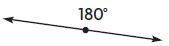Options:
a. acute
b. obtuse
c. right
d. straight

Explanation:
180° is nothing but a straight angle.
Thus the correct answer is option D.

Question 2.
How many degrees are in an angle that turns through $$\frac{1}{4}$$ of a circle?
Options:
a. 45°
b. 90°
c. 180°
d. 270°

Explanation:
The complete angle of the circle is 360°.
$$\frac{1}{4}$$ × 360° = 90°
Thus the correct answer is option B.

Spiral Review

Question 3.
Mae bought 15 football cards and 18 baseball cards. She separated them into 3 equal groups. How many sports cards are in each group?
Options:
a. 5
b. 6
c. 11
d. 12

Explanation:
Given that,
Mae bought 15 football cards and 18 baseball cards. She separated them into 3 equal groups.
The total cards = 15 + 18 = 33 cards
Divide 33 cards into 3 equal groups
33/3 = 11
Thus the correct answer is option C.

Question 4.
Each part of a race is $$\frac{1}{10}$$ mile long. Marsha finished 5 parts of the race. How far did Marsha race?
Options:
a. $$\frac{1}{10}$$ mile
b. $$\frac{5}{12}$$ mile
c. $$\frac{1}{2}$$ mile
d. 5 $$\frac{1}{10}$$ miles

Answer: $$\frac{1}{2}$$ mile

Explanation:
Each part of a race is $$\frac{1}{10}$$ mile long. Marsha finished 5 parts of the race.
We have to divide $$\frac{1}{10}$$ into 5 parts.
$$\frac{1}{10}$$ ÷ 5 = $$\frac{1}{2}$$ mile
Thus the correct answer is option C.

Question 5.
Jeff said his city got $$\frac{11}{3}$$ inches of snow. Which shows this fraction written as a mixed number?
Options:
a. 3 $$\frac{2}{3}$$
b. 3 $$\frac{1}{3}$$
c. 2 $$\frac{2}{3}$$
d. 1 $$\frac{2}{3}$$

Answer: 3 $$\frac{2}{3}$$

Explanation:
Jeff said his city got $$\frac{11}{3}$$ inches of snow.
Convert from improper fraction into the mixed fraction.
$$\frac{11}{3}$$ = 3 $$\frac{2}{3}$$
Thus the correct answer is option A.

Question 6.
Amy ran $$\frac{3}{4}$$ mile. Which decimal shows how many miles she ran?
Options:
a. 0.25 mile
b. 0.34 mile
c. 0.5 mile
d. 0.75 mile

Explanation:
Given,
Amy ran $$\frac{3}{4}$$ mile.
The decimal form of $$\frac{3}{4}$$ is 0.75
She ran 0.75 miles.
Thus the correct answer is option D.

### Common Core – Angles – Page No. 211

Measure and Draw Angles

Use a protractor to find the angle measure.

Question 1.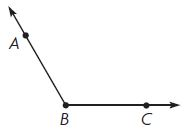m∠ABC= 120°

Question 2.m∠MNP = _____°

By using the protractor we can measure the angle. m∠MNP = 90°

Question 3.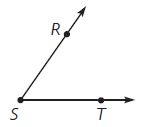m∠RST = _____°

By using the protractor we can measure the angle m∠RST is 55°

Use a protractor to draw the angle.

Question 4.
40°

Question 5.
170°

Draw an example of each. Label the angle with its measure.

Question 6.
a right angleQuestion 7.
an acute angleProblem Solving

The drawing shows the angles a stair tread makes with a support board along a wall. Use your protractor to measure the angles.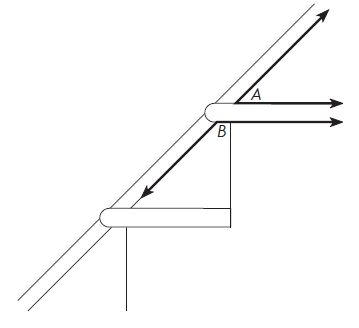Question 8.
What is the measure of ∠A?
_____°

By using the protractor we can measure the angle ∠A = 45°

Question 9.
What is the measure of ∠B?
_____°

By using the protractor we can measure the angle ∠B = 135°

### Common Core – Angles – Page No. 212

Lesson Check

Question 1.
What is the measure of ∠ABC?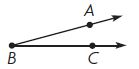Options:
a. 15°
b. 25°
c. 155°
d. 165°

With the help of the protractor, we can measure the ∠ABC = 15°
The correct answer is option A.

Question 2.
What is the measure of ∠XYZ?Options:
a. 20°
b. 30°
c. 150°
d. 160°

With the help of the protractor, we can measure the ∠XYZ = 150°
The correct answer is option C.

Spiral Review

Question 3.
Derrick earned $1,472 during the 4 weeks he had his summer job. If he earned the same amount each week, how much did he earn each week? Options: a.$360
b. $368 c.$3,680
d. $5,888 Answer:$368

Explanation:
Derrick earned $1,472 during the 4 weeks he had his summer job. Divide 1472 by 4 1472/4 =$368
Therefore he earned $368 each week. Thus the correct answer is option B. Question 4. Arthur baked 1 $$\frac{7}{12}$$ dozen muffins. Nina baked 1 $$\frac{1}{12}$$ dozen muffins. How many dozen muffins did they bake in all? Options: a. 3 $$\frac{2}{3}$$ b. 2 $$\frac{2}{3}$$ c. 2 $$\frac{1}{2}$$ d. $$\frac{6}{12}$$ Answer: 2 $$\frac{2}{3}$$ Explanation: Arthur baked 1 $$\frac{7}{12}$$ dozen muffins. Nina baked 1 $$\frac{1}{12}$$ dozen muffins. Add both the fraction 1 $$\frac{7}{12}$$ + 1 $$\frac{1}{12}$$ First add the whole numbers 1 + 1 = 2 $$\frac{7}{12}$$ + $$\frac{1}{12}$$ = $$\frac{8}{12}$$ 2 $$\frac{8}{12}$$ = 2 $$\frac{2}{3}$$ Thus the correct answer is option B. Question 5. Trisha drew the figure below. What figure did she draw?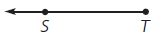Options: a. line segment ST b. ray ST c. ray TS d. line TS Answer: ray TS The name of the figure Trisha drew is ray TS. The correct answer is option C. Question 6. Which describes the turn shown by the angle?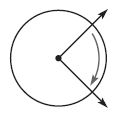Options: a. 1 full turn clockwise b. $$\frac{3}{4}$$ turn clockwise c. $$\frac{1}{2}$$ turn clockwise d. $$\frac{1}{4}$$ turn clockwise Answer: $$\frac{1}{4}$$ turn clockwise Explanation: The circle made a turn clockwise with a fraction $$\frac{1}{4}$$. Thus the correct answer is option D. ### Common Core – Angles – Page No. 213 Join and Separate Angles Add to find the measure of the angle. Write an equation to record your work. Question 1.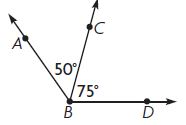50°+75°=125° m∠ABD=125° Question 2._____° + _____° = _____° ; m∠FGJ = _____° Answer: 160° Explanation: m∠FGH = 140° m∠HGJ = 20° m∠FGJ = 140° + 20° = 160° Question 3._____° + _____° + _____° = _____° ; m∠KLN = _____° Answer: 165° Explanation: m∠KLM = 30° m∠MLP = 90° m∠PLN = 45° m∠KLN = 30° + 90° + 45° = 165° Use a protractor to find the measure of each angle in the circle.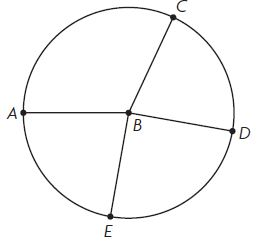Question 4. m∠ABC = _____° Answer: 115° By using the protractor we can measure m∠ABC = 115° Question 5. m∠DBE = _____° Answer: 90° By using the protractor we can measure m∠DBE = 90° Question 6. m∠CBD = _____° Answer: 75° By using the protractor we can measure m∠CBD = 75° Question 7. m∠EBA = _____° Answer: 80° By using the protractor we can measure m∠EBA = 80° Question 8. Write the sum of the angle measures as an equation. _____° + _____° + _____° + _____° = _____° Answer: 115° + 75° + 90° + 80° = 360° Explanation: m∠ABC + m∠DBE + m∠CBD + m∠EBA 115° + 75° + 90° + 80° = 360° Problem Solving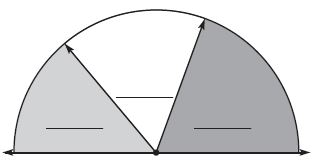Question 9. Ned made the design at the right. Use a protractor. Find and write the measure of each of the 3 angles. _____° ; _____° ; _____° ; Answer: 50°; 60°; 70° By using the protractor we can measure each of the 3 angles i.e, 50°; 60°; 70° Question 10. Write an equation to find the measure of the total angle. _____° + _____° + _____° = _____° Answer: 50° + 60° + 70° =180° Explanation: Add all the three angles = 50° + 60° + 70° =180° ### Common Core – Angles – Page No. 214 Lesson Check Question 1. What is the measure of m∠WXZ?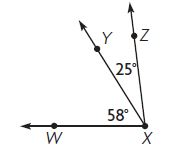Options: a. 32° b. 83° c. 88° d. 97° Answer: 83° Explanation: m∠WXY = 58° m∠ZXY = 25° m∠WXZ = m∠WXY + m∠ZXY m∠WXZ = 58° + 25° m∠WXZ = 83° Thus the correct answer is option B. Question 2. Which equation can you use to find the m∠MNQ?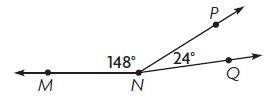Options: a. 148° – 24° = ■ b. 148° × 24° = ■ c. 148° ÷ 24° = ■ d. 148° + 24° = ■ Answer: 148° + 24° = ■ Explanation: m∠MNQ = m∠MNP + m∠PNQ m∠MNP + m∠PNQ = 148° + 24° m∠MNQ = ■ 148° + 24° = ■ Thus the correct answer is option D. Spiral Review Question 3. Joe bought 6 packages of envelopes. Each package contains 125 envelopes. How many envelopes did he buy? Options: a. 750 b. 723 c. 720 d. 650 Answer: 750 Explanation: Given, Joe bought 6 packages of envelopes. Each package contains 125 envelopes. Multiply the number of packages and number of envelopes = 6 × 125 = 750 Thus the correct answer is option A. Question 4. The Lake Trail is $$\frac{3}{10}$$ mile long and the Rock Trail is $$\frac{5}{10}$$ long. Bill hiked each trail once. How many miles did he hike in all? Options: a. $$\frac{1}{5}$$ mile b. $$\frac{4}{10}$$ mile c. $$\frac{1}{2}$$ mile d. $$\frac{8}{10}$$ mile Answer: $$\frac{8}{10}$$ mile Explanation: The Lake Trail is $$\frac{3}{10}$$ mile long and the Rock Trail is $$\frac{5}{10}$$ long. $$\frac{3}{10}$$ + $$\frac{5}{10}$$ = $$\frac{8}{10}$$ mile Thus the correct answer is option D. Question 5. Ron drew a quadrilateral with 4 right angles and 4 sides with the same length. Which best describes the figure he drew? Options: a. square b. rhombus c. trapezoid d. parallelogram Answer: square Explanation: A quadrilateral with 4 right angles and 4 sides with the same length is known as a square. Question 6. How many degrees are in an angle that turns through $$\frac{3}{4}$$ of a circle? Options: a. 45° b. 90° c. 180° d. 270° Answer: 270° Explanation: $$\frac{3}{4}$$ of a circle is 3/4 × 360° = 3 × 90° = 270° Thus the correct answer is option D. ### Common Core – Angles – Page No. 215 Problem Solving Unknown Angle Measures Solve each problem. Draw a diagram to help. Question 1.Question 2. An artist is cutting a piece of metal as shown. What is the angle measure of the piece left over?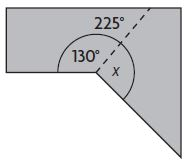x = _____° Answer: 95° Explanation: x + 130° = 225 x = 225° – 130° x = 95° Question 3. Joan has a piece of material for making a costume. She needs to cut it as shown. What is the angle measure of the piece left over?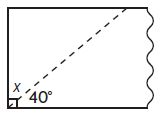x = _____° Answer: 50° Explanation: x + 40° = 90° x = 90° – 40° x = 50° ### Common Core – Angles – Page No. 216 Lesson Check Question 1. Angelo cuts a triangle from a sheet of paper as shown. What is the measure of ∠x in the triangle?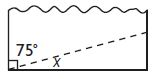Options: a. 15° b. 25° c. 75° d. 105° Answer: 15° Explanation: The above figure is a right triangle. x + 75° = 90° x = 90° – 75° x = 15° Thus the correct answer is option A. Question 2. Cindy cuts a piece of wood as shown. What is the angle measure of the piece left over?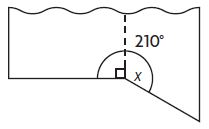Options: a. 30° b. 90° c. 120° d. 150° Answer: 120° Explanation: x + 90° = 210° x = 210° – 90 x = 120° Thus the correct answer is option C. Spiral Review Question 3. Tyronne worked 21 days last month. He earned$79 each day. How much did Tyronne earn last month?
Options:
a. $869 b.$948
c. $1,659 d.$2,169

Answer: $1,659 Explanation: Given that, Tyronne worked 21 days last month. He earned$79 each day.
21 × $79 =$1659
Thus the correct answer is option C.

Question 4.
Meg inline skated for $$\frac{7}{10}$$ mile. Which shows this distance written as a decimal?
Options:
a. 0.07 mile
b. 0.1 mile
c. 0.7 mile
d. 7.1 miles

Explanation:
Meg inline skated for $$\frac{7}{10}$$ mile.
The decimal form of $$\frac{7}{10}$$ is 0.7 mile.
Thus the correct answer is option C.

Question 5.
Kerry ran $$\frac{3}{4}$$ mile. Sherrie ran $$\frac{1}{2}$$ mile. Marcie ran $$\frac{2}{3}$$ mile. Which list orders the friends from least to greatest distance
run?
Options:
a. Kerry, Sherrie, Marcie
b. Kerry, Marcie, Sherrie
c. Sherrie, Kerry, Marcie
d. Sherrie, Marcie, Kerry

Explanation:
Kerry ran $$\frac{3}{4}$$ mile. Sherrie ran $$\frac{1}{2}$$ mile. Marcie ran $$\frac{2}{3}$$ mile.
Put the fractions from least to greatest.
$$\frac{1}{2}$$, $$\frac{2}{3}$$, $$\frac{3}{4}$$
Thus the correct answer is option D.

Question 6.
What is the measure of m∠ABC?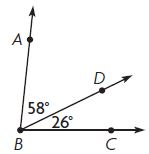Options:
a. 32°
b. 84°
c. 88°
d. 94°

Explanation:
m∠ABC = m∠ABD + m∠DBC
m∠ABC = 58° + 26°
m∠ABC = 84°
Thus the correct answer is option B.

### Common Core – Angles – Page No. 217

Lesson 11.1

Tell whether the angle on the circle shows $$\frac{1}{4}, \frac{1}{2}, \frac{3}{4}$$, or 1 full turn clockwise or counterclockwise.

Question 1.$$\frac{□}{□}$$

Answer: $$\frac{1}{4}$$

Explanation:
The angle on the above circle shows $$\frac{1}{4}$$ turn counterclockwise.

Question 2._____

The angle on the above circle shows 1 full turn clockwise.

Question 3.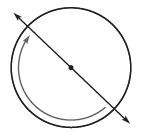$$\frac{□}{□}$$

Answer: $$\frac{1}{2}$$
The angle on the above circle shows $$\frac{1}{2}$$ turn clockwise.

Lesson 11.2

Tell the measure of the angle in degrees.

Question 4.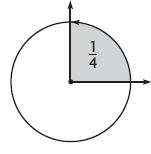_____

The complete angle of the circle = 360°
The fraction of the shaded part is 1/4
1/4 × 360° = 90°

Question 5.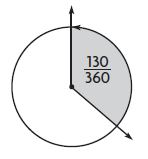_____

The complete angle of the circle = 360°
The fraction of the shaded part is 130/360
130/360 × 360 = 130°

Question 6._____

Explanation:
The complete angle of the circle = 360°
The fraction of the shaded part is 3/4
3/4 × 360° = 270°

Classify the triangle. Write acute, obtuse, or right.

Question 7.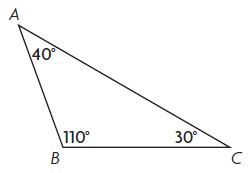_____

Explanation:
An obtuse angle has a measurement greater than 90 degrees but less than 180 degrees. The above angle is 110° which is greater than 90° and less than 180°. Thus the angle of the above figure is an obtuse angle.

Question 8._____

Explanation:
The acute angle is the small angle which is less than 90°. The above angle is 60° which is less than 90°. Thus the above angle is an acute angle.

Question 9._____

Explanation:
In geometry and trigonometry, a right angle is an angle of exactly 90°, corresponding to a quarter turn.

### Common Core – Angles – Page No. 218

Lesson 11.3

Question 1.
Use a protractor to find the angle measure.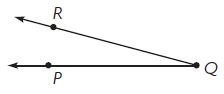m ∠PQR = _____°

By using the protractor we can measure the angle m ∠PQR = 15°

Question 2.
Use a protractor to draw an angle with the measure 72º.

Lesson 11.4

Add to find the measure of the angle. Write an equation to record your work.

Question 3.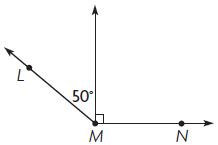m ∠NML = _____°

Explanation:
m ∠NML = m ∠LMX + m ∠NMX
m ∠NML = 50° + 90°
m ∠NML = 140°

Question 4.m ∠UTS = _____°

Explanation:
m ∠UTS = m ∠STX + m ∠UTX
m ∠UTS = 25° + 30°
m ∠UTS = 55°

Question 5.m ∠HGF = _____°

Explanation:
m ∠HGF = m ∠HGX + m ∠HGY + m ∠FGY
m ∠HGF = 45° + 50° + 70° = 165°
m ∠HGF = 165°

Lesson 11.5

Use the diagram for 1–2.Question 6.
Luke is cutting a board to make a trapezoid for a project. What is the angle measure of the piece left over after Cut A?
x = _____°

Explanation:
By seeing the above figure we can find Cut A.
x + 55° = 90°
x = 90° – 55°
x = 35°

Question 7.
What is the angle measure of the piece left over after Cut B?
y = _____°